# Linear representation theory of general linear group:GL(2,3)

This article gives specific information, namely, linear representation theory, about a particular group, namely: general linear group:GL(2,3).
View linear representation theory of particular groups | View other specific information about general linear group:GL(2,3)

This article describes the linear representation theory (in characteristic zero and other characteristics excluding 2,3) of general linear group:GL(2,3), which is the general linear group of degree two over field:F3.

## Summary

Item Value
degrees of irreducible representations over a splitting field 1,1,2,2,2,3,3,4
maximum: 4, lcm: 12, number: 8, sum of squares: 48
ring generated by character values (characteristic zero)$\mathbb{Z}[\sqrt{-2}]$, same as$\mathbb{Z}[t]/(t^2 + 2)$
field generated by character values (characteristic zero)$\mathbb{Q}(\sqrt{-2})$, same as$\mathbb{Q}[t]/(t^2 + 2)$

## Irreducible representations

### Interpretation as general linear group of degree two

The group is a general linear group of degree two over field:F3. Compare with linear representation theory of general linear group of degree two over a finite field.

Description of collection of representations Parameter for describing each representation How the representation is described Degree of each representation (generic$q$) Degree of each representation ($q = 3$) Number of representations (generic$q$) Number of representations ($q = 3$) Sum of squares of degrees (generic$q$) Sum of squares of degrees ($q = 3$)
One-dimensional, factor through the determinant map a homomorphism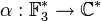$\alpha: \mathbb{F}_3^\ast \to \mathbb{C}^\ast$$x \mapsto \alpha(\det x)$ 1 1$q - 1$ 2$q - 1$ 2
Unclear a homomorphism$\varphi:\mathbb{F}_{9}^\ast \to \mathbb{C}^\ast$ unclear$q - 1$ 2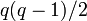$q(q - 1)/2$ 3$q(q - 1)^3/2$ 12
Tensor product of one-dimensional representation and the nontrivial component of permutation representation of$GL_2$ on the projective line over$\mathbb{F}_3$ a homomorphism$\alpha: \mathbb{F}_3^\ast \to \mathbb{C}^\ast$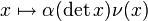$x \mapsto \alpha(\det x)\nu(x)$ where$\nu$ is the nontrivial component of permutation representation of$GL_2$ on the projective line over$\mathbb{F}_3$$q$ 3$q - 1$ 2$q^2(q - 1)$ 18
Induced from one-dimensional representation of Borel subgroup Both distinct representations$\alpha, \beta$ homomorphisms$\mathbb{F}_3^\ast \to \mathbb{C}^\ast$ Induced from the following representation of the Borel subgroup:$\begin{pmatrix} a & b \\ 0 & d \\\end{pmatrix} \mapsto \alpha(a)\beta(d)$$q + 1$ 4$(q - 1)(q - 2)/2$ 1$(q+1)^2(q-1)(q-2)/2$ 16
Total NA NA NA NA$q^2 - 1$ 8$q^4 - q^3 - q^2 + q$ 48

## Character table

FACTS TO CHECK AGAINST (for characters of irreducible linear representations over a splitting field):
Orthogonality relations: Character orthogonality theorem | Column orthogonality theorem
Separation results (basically says rows independent, columns independent): Splitting implies characters form a basis for space of class functions|Character determines representation in characteristic zero
Numerical facts: Characters are cyclotomic integers | Size-degree-weighted characters are algebraic integers
Character value facts: Irreducible character of degree greater than one takes value zero on some conjugacy class| Conjugacy class of more than average size has character value zero for some irreducible character | Zero-or-scalar lemma

In the table below, we denote by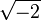$\sqrt{-2}$ a fixed square root of -2.

Representation/conjugacy class representative and size$\begin{pmatrix} 1 & 0 \\ 0 & 1 \\\end{pmatrix}$ (size 1)$\begin{pmatrix} -1 & 0 \\ 0 & -1 \\\end{pmatrix}$ (size 1)$\begin{pmatrix} 0 & 1 \\ -1 & 0 \\\end{pmatrix}$ (size 6)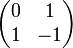$\begin{pmatrix} 0 & 1 \\ 1 & -1 \\\end{pmatrix}$ (size 6)$\begin{pmatrix} 0 & 1 \\ 1 & 1 \\\end{pmatrix}$ (size 6)$\begin{pmatrix} 1 & 1 \\ 0 & 1 \\\end{pmatrix}$ (size 8)$\begin{pmatrix} -1 & 1 \\ 0 & -1 \\\end{pmatrix}$ (size 8)$\begin{pmatrix} 1 & 0 \\ 0 & -1 \\\end{pmatrix}$ (size 12)
trivial 1 1 1 1 1 1 1 1
nontrivial one-dimensional 1 1 1 -1 -1 1 1 -1
two-dimensional (unclear) 2 2 2 0 0 -1 -1 0
two-dimensional (unclear) 2 -2 0$\sqrt{-2}$$-\sqrt{-2}$ -1 1 0
two-dimensional (unclear) 2 -2 0$-\sqrt{-2}$$\sqrt{-2}$ -1 1 0
three-dimensional, factors through standard representation of symmetric group:S4 3 3 -1 -1 -1 0 0 1
three-dimensional, factors through tensor product of standard and sign representations of$S_4$ 3 3 -1 1 1 0 0 -1
four-dimensional, induced from one-dimensional representation of Borel subgroup 4 -4 0 0 0 1 -1 0

## GAP implementation

### Degrees of irreducible representations

The degrees of irreducible representations can be computed using GAP's CharacterDegrees function, as follows:

gap> CharacterDegrees(GL(2,3));
[ [ 1, 2 ], [ 2, 3 ], [ 3, 2 ], [ 4, 1 ] ]

### Character table

The character table can be computed using GAP's CharacterTable function, as follows:

gap> Irr(CharacterTable(GL(2,3)));
[ Character( CharacterTable( GL(2,3) ), [ 1, 1, 1, 1, 1, 1, 1, 1 ] ),
Character( CharacterTable( GL(2,3) ), [ 1, 1, 1, 1, 1, -1, -1, -1 ] ),
Character( CharacterTable( GL(2,3) ), [ 2, -1, 2, -1, 2, 0, 0, 0 ] ),
Character( CharacterTable( GL(2,3) ), [ 2, 1, -2, -1, 0, -E(8)-E(8)^3,
E(8)+E(8)^3, 0 ] ), Character( CharacterTable( GL(2,3) ),
[ 2, 1, -2, -1, 0, E(8)+E(8)^3, -E(8)-E(8)^3, 0 ] ),
Character( CharacterTable( GL(2,3) ), [ 3, 0, 3, 0, -1, 1, 1, -1 ] ),
Character( CharacterTable( GL(2,3) ), [ 3, 0, 3, 0, -1, -1, -1, 1 ] ),
Character( CharacterTable( GL(2,3) ), [ 4, -1, -4, 1, 0, 0, 0, 0 ] ) ]

A visual display of the character table can be achieved as follows:

gap> Display(CharacterTable(GL(2,3)));
CT1

2  4  1  4  1  3  3  3  2
3  1  1  1  1  .  .  .  .

1a 6a 2a 3a 4a 8a 8b 2b

X.1     1  1  1  1  1  1  1  1
X.2     1  1  1  1  1 -1 -1 -1
X.3     2 -1  2 -1  2  .  .  .
X.4     2  1 -2 -1  .  A -A  .
X.5     2  1 -2 -1  . -A  A  .
X.6     3  .  3  . -1  1  1 -1
X.7     3  .  3  . -1 -1 -1  1
X.8     4 -1 -4  1  .  .  .  .

A = -E(8)-E(8)^3
= -Sqrt(-2) = -i2

### Irreducible representations

The irreducible representations of$GL(2,3)$ can be computed using GAP's GAP:IrreducibleRepresentations function, as follows:

gap> IrreducibleRepresentations(GL(2,3));
[ CompositionMapping( [ (4,7)(5,8)(6,9), (2,7,6)(3,4,8) ] -> [ [ [ 1 ] ], [ [ 1 ] ] ], <action isomorphism> ), CompositionMapping( [ (4,7)(5,8)(6,9), (2,7,6)(3,4,8)
] -> [ [ [ -1 ] ], [ [ 1 ] ] ], <action isomorphism> ), CompositionMapping( [ (4,7)(5,8)(6,9), (2,7,6)(3,4,8) ] -> [ [ [ 0, E(3) ], [ E(3)^2, 0 ] ],
[ [ E(3), 0 ], [ 0, E(3)^2 ] ] ], <action isomorphism> ), CompositionMapping( [ (4,7)(5,8)(6,9), (2,7,6)(3,4,8) ] ->
[ [ [ -1/2*E(24)^11-1/2*E(24)^17, -1/2*E(24)-E(24)^11-E(24)^17-1/2*E(24)^19 ], [ -1/2*E(8)-1/2*E(8)^3, 1/2*E(24)^11+1/2*E(24)^17 ] ],
[ [ E(3), E(3) ], [ 0, E(3)^2 ] ] ], <action isomorphism> ), CompositionMapping( [ (4,7)(5,8)(6,9), (2,7,6)(3,4,8) ] ->
[ [ [ E(24)+E(24)^19, -1 ], [ -E(3)+E(3)^2, -E(24)-E(24)^19 ] ], [ [ E(3)+2*E(3)^2, E(8)+E(8)^3 ], [ -E(24)-E(24)^19, -E(3)^2 ] ] ], <action isomorphism> ),
CompositionMapping( [ (4,7)(5,8)(6,9), (2,7,6)(3,4,8) ] -> [ [ [ 0, 0, 1 ], [ 0, -1, 0 ], [ 1, 0, 0 ] ], [ [ 1, 0, 0 ], [ 0, 0, 1 ], [ 1, -1, -1 ] ] ],
<action isomorphism> ), CompositionMapping( [ (4,7)(5,8)(6,9), (2,7,6)(3,4,8) ] -> [ [ [ 0, 1, 0 ], [ 1, 0, 0 ], [ 0, 0, 1 ] ],
[ [ 1, 0, 0 ], [ -1, -1, -1 ], [ 0, 1, 0 ] ] ], <action isomorphism> ), CompositionMapping( [ (4,7)(5,8)(6,9), (2,7,6)(3,4,8) ] ->
[ [ [ 0, 0, -E(3), 0 ], [ 0, 0, -E(3), -E(3) ], [ -E(3)^2, 0, 0, 0 ], [ E(3)^2, -E(3)^2, 0, 0 ] ],
[ [ -E(3)^2, E(3)^2, 0, 0 ], [ -E(3)^2, 0, 0, 0 ], [ 0, 0, E(3)^2, 0 ], [ 0, 0, 1, 1 ] ] ], <action isomorphism> ) ]